### Six Sevenths of a Fraction Pie

Six sevenths of a circle.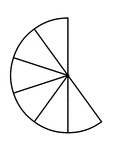### Six Tenths of a Fraction Pie

Six tenths of a circle.### Six Twelfths of a Fraction Pie

Six twelfths of a circle.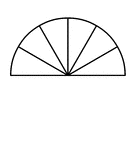### Six Twelfths of a Fraction Pie

Six twelfths of a circle.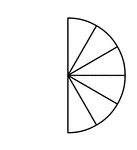### Six Twelfths of a Fraction Pie

Six twelfths of a circle.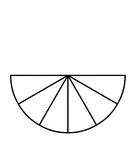### Six Twelfths of a Fraction Pie

Six twelfths of a circle.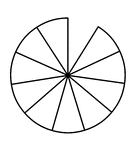### Ten Elevenths of a Fraction Pie

Ten elevenths of a circle.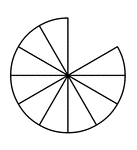### Ten Twelfths of a Fraction Pie

Ten twelfths of a circle.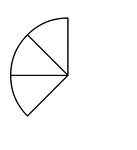### Three Eighths of a Fraction Pie

Three eighths of a circle.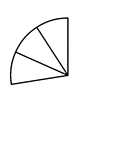### Three Elevenths of a Fraction Pie

Three elevenths of a circle.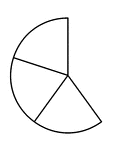### Three Fifths of a Fraction Pie

Three fifths of a circle.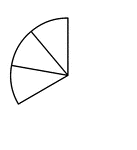### Three Ninths of a Fraction Pie

Three ninths of a circle.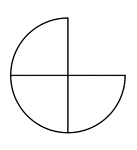### Three Quarters of a Fraction Pie

Three quarters of a circle.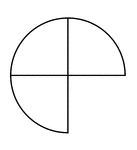### Three Quarters of a Fraction Pie

Three quarters of a circle.### Three Quarters of a Fraction Pie

Three quarters of a circle.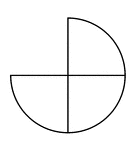### Three Quarters of a Fraction Pie

Three quarters of a circle.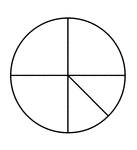### Three Quarters and Two Eighths of a Pie Fraction

A circle subdivided into three quarters and two eighths.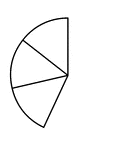### Three Sevenths of a Fraction Pie

Three sevenths of a circle.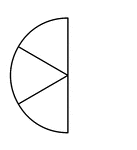### Three Sixths of a Fraction Pie

Three sixths of a circle.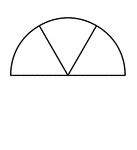### Three Sixths of a Fraction Pie

Three sixths of a circle.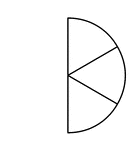### Three Sixths of a Fraction Pie

Three sixths of a circle.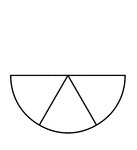### Three Sixths of a Fraction Pie

Three sixths of a circle.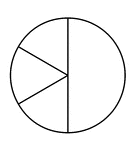### Three Sixths and One Half of a Pie Fraction

A circle subdivided into three sixths and one half.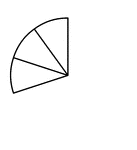### Three Tenths of a Fraction Pie

Three tenths of a circle.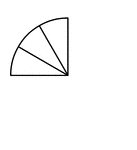### Three Twelfths of a Fraction Pie

Three twelfths of a circle.### Three Twelfths of a Fraction Pie

Three twelfths of a circle.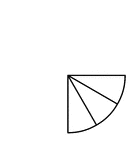### Three Twelfths of a Fraction Pie

Three twelfths of a circle.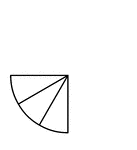### Three Twelfths of a Fraction Pie

Three twelfths of a circle.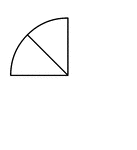### Two Eighths of a Fraction Pie

Two eighths of a circle.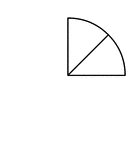### Two Eighths of a Fraction Pie

Two eighths of a circle.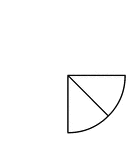### Two Eighths of a Fraction Pie

Two eighths of a circle.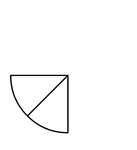### Two Eighths of a Fraction Pie

Two eighths of a circle.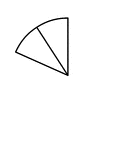### Two Elevenths of a Fraction Pie

Two elevenths of a circle.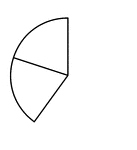### Two Fifths of a Fraction Pie

Two fifths of a circle.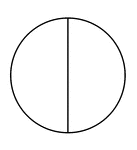### Two Halves of a Fraction Pie

A circle divided in half vertically.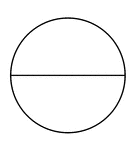### Two Halves of a Fraction Pie

A circle divided in half horizontally.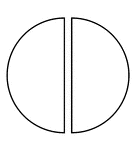### Two Halves of a Fraction Pie

A circle divided in half vertically separated.### Two Halves of a Fraction Pie

A circle divided in half horizontally separated.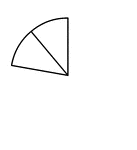### Two Ninths of a Fraction Pie

Two ninths of a circle.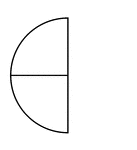### Two Quarters of a Fraction Pie

Two quarters of a circle.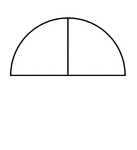### Two Quarters of a Fraction Pie

Two quarters of a circle.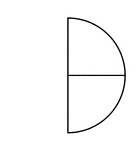### Two Quarters of a Fraction Pie

Two quarters of a circle.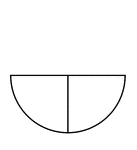### Two Quarters of a Fraction Pie

Two quarters of a circle.### Two Quarters and One Half of a Pie Fraction

A circle subdivided into two quarters and one half.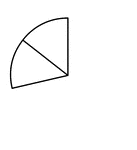### Two Sevenths of a Fraction Pie

Two sevenths of a circle.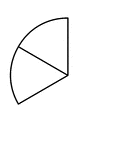### Two Sixths of a Fraction Pie

Two sixths of a circle.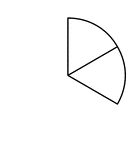### Two Sixths of a Fraction Pie

Two sixths of a circle.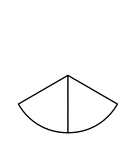### Two Sixths of a Fraction Pie

Two sixths of a circle.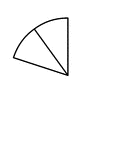### Two Tenths of a Fraction Pie

Two tenths of a circle.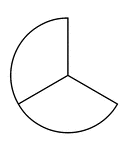### Two Thirds of a Fraction Pie

Two thirds of a circle.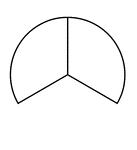### Two Thirds of a Fraction Pie

Two thirds of a circle.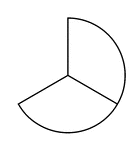### Two Thirds of a Fraction Pie

Two thirds of a circle.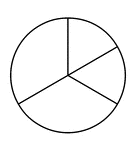### Two Thirds and Two Sixths of a Pie Fraction

A circle subdivided into two thirds and two sixths.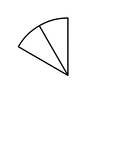### Two Twelfths of a Fraction Pie

Two twelfths of a circle.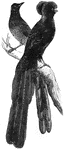Native to New Guinea, the pie of paradise is renowned for its brilliant plumage.### Pirate Sitting On Barrel Eating Pie

An illustration of a pirate sitting on a barrel eating a slice of pie.### Simple Simon

Simple Simon met a pie-man going to the fair.